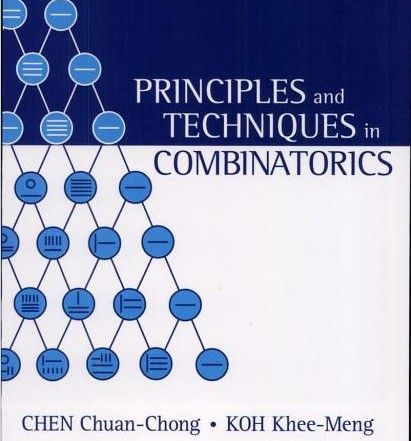## Thursday, 24 December 2009

### SPLITTING FTA FUNCTIONS (III)

PILTZ DIVISOR FUNCTIONS (2)THEOREM-2:

$latex \tau_{j+k}(n)=\sum_{d|n}^{}{\tau_{j}(d)\cdot\tau_{k}\left(\frac{n}{d}\right)}$

Proof:

The Piltz Divisor functions are multiplicative, so it is only necessary to prove the case $latex p^{\alpha}$

In the previous post we saw how:

$latex \tau_{k}(p^\alpha)=\binom{\alpha+k-1}{k-1}$

And if we apply $latex p^\alpha$ to second member of the problem identity, then:

$latex f(p^\alpha)=\sum_{d|p^\alpha}^{}{\tau_{j}(d)\cdot\tau_{k}\left(\frac{p^\alpha}{d}\right)}=\sum_{i=0}^{\alpha}{\tau_{j}(p^i)\cdot\tau_{k}(p^{\alpha-i})=\sum_{i=0}^{\alpha}{\binom{i+j-1}{j-1}\binom{\alpha-i+k-1}{k-1}=$

$latex =\binom{\alpha+j+k-1}{j+k-1}=\tau_{j+k}(p^\alpha)$

This proof seems easy except for the binomial identity step:

$latex \sum_{i=0}^{\alpha}{\binom{i+j-1}{j-1}\binom{\alpha-i+k-1}{k-1}=\binom{\alpha+j+k-1}{j+k-1}$

After several unfruitful tries to prove it, due to my lack of mathematical skills, I resigned myself to look for information about this problem on the bibliography, this formula look very close to the one found on reference  or , but with an additional variable, and after many reviews, I was glad to find a combinatorial version of the proof into the book of Chuan Chong Chen and Khee-Meng Koh 

This proof solved the problem, but it let me very much unsatisfied, and I begun to rethink about this topic again.

This problem is about Number Theory and not Combinatorics, and I had to revise the first lesson about the properties of Dirichlet Product:

Dirichlet´s functional convolution is associative: we can put the brackets wherever we want, so:

$latex \tau_{j+k}(n)=(\underbrace{I_{0}(n)*...*I_{0}(n)}_{j}) *( \underbrace{I_{0}(n)*...*I_{0}(n)}_{k})=\tau_{j}(n)*\tau_{k}(n)$

This simple line proves this trivial property of the Piltz functions that I pedanticly considered as a Theorem, and it proves, as a tip, the binomial formula. The readers of this blog (if any) may forgive me.

But after all of this mess, I´ve learned many interesting things:

1) Number Theory counts with powerful mathematical tools than can be used for many unexpected purposes, just to mention the relationship between the Piltz functions and the Jacobi polynomials.
2) The properties of arithmetical functions can be used to get elegant proofs for binomial identities. (This is the opposite way that the one I took).
3) In my effort to deal with binomial identities, I discovered some formulas for determinants of matrices with binomial coefficients. (Well, there´s many articles about this topic, but I worked without previous knowledge of them). Anyhow, I haven´t found this formula somewhere but here.

References:

-Matthew Hubbard and Tom Roby - Pascal's Triangle From Top To Bottom -Catalog #: 31000005
-Ronald L. Graham, Donald E. Knuth, and Oren Patashnik (Reading, Massachusetts: Addison-Wesley, 1994 - Concrete Mathematics - Identity (5.26)
-Chuan Chong Chen,Khee-Meng Koh - Principles and techniques in combinatorics page 88-Example 2.6.2-Shortest Routes in Rectangular Grid.